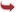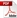International Journal of Scientific and Research Publications

#### IJSRP, Volume 3, Issue 12, December 2013 Edition [ISSN 2250-3153]Fuzzy Multiple Domination
G.Nirmala, M.Sheela
Abstract: The fuzzy domination number (G) of the fuzzy graph G is the minimum cardinality taken over all fuzzy minimal dominating set of G. The minimum cardinality of a fuzzy k-dominating set is called the fuzzy k-dominating number k(G) .The maximum incident degree of a fuzzy graph is (G).In this paper we prove some theorems that relate the parameters(G),k(G),(G).
[VIEW FULL PAPER][DOWNLOAD]

Reference this Research Paper (copy & paste below code):

G.Nirmala, M.Sheela (2018); Fuzzy Multiple Domination; Int J Sci Res Publ 3(12) (ISSN: 2250-3153). http://www.ijsrp.org/research-paper-1213.php?rp=P242043